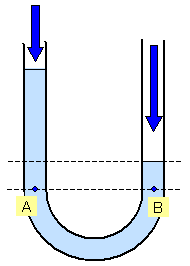# The U tube manometerIn the left diagram a U tube is partly filled with liquid. The pressure of the air on both the open ends is the same, the pressure at the points A and B must be the same (same level in the liquid) and so the levels of the top of each limb of the U tube are equal.

In the right hand diagram the U tube is still partly filled with liquid but this time someone blows into the right hand open end. The pressure at the points A and B must still be the same (same level in the liquid) and so the height of the column of liquid on the right is less than that on the left.

The pressure at A is due to the ordinary air pressure plus the height of the column above A and the pressure at B is the higher air pressure plus the liquid column above B.

© Keith Gibbs 2011